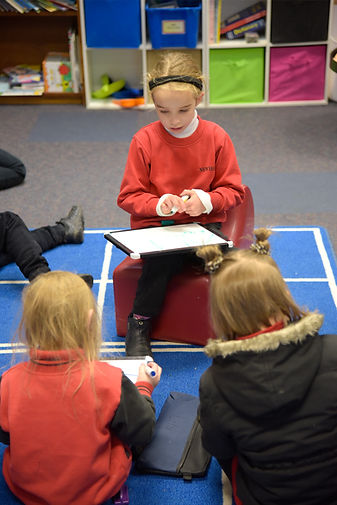# Numeracy

What a Maths lesson looks like:

Everyday students get clear, teacher-led instruction about the maths skills they’re learning. Throughout the lesson, teachers introduce skills and algorithms for the students to follow, foster discussion about different ways to find answers using maths language and let kids explore creative solutions.

Maths lessons focus on building students conceptual knowledge with hands-on activities, oral practice, and math games that link Maths to daily life.  Maths games aren’t just filler or fun – they’re threaded throughout the daily lessons an considered a big part of your child’s learning.

Number, measurement and geometry, statistics and probability are the aspects covered by the curriculum.  The Mathematics curriculum focuses on developing mathematical understanding, fluency, reasoning, modelling and problem solving.  These capabilities enable students to respond to familiar and unfamiliar situations by employing mathematics to make informed decisions and solve problems efficiently.

The curriculum ensures that the links between the various components of mathematics, as well as the relationship between mathematics and other disciplines, are made clear.